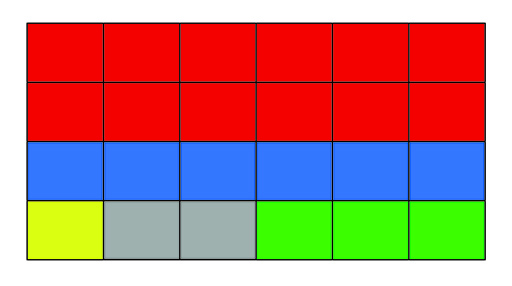Teaching mathematics

Start this free course now. Just create an account and sign in. Enrol and complete the course for a free statement of participation or digital badge if available.

Free course

# 2.3 Adding and subtracting fractions

Learners are often told that the denominator in the fraction denotes what kind of a fraction it is (halves, thirds, etc.) and that it is only possible to add or subtract the same types of fraction, i.e. the denominator has to be the same number. This is where the difficulty lies as learners struggle to find out which number should be the common denominator and what needs to be done to change each fraction so that they do have the same denominator. Several rules and procedures exist to help learners to add and subtract fractions but, since the conceptual basis is poorly understood, learners typically do not remember the rules and procedures. This often leads to fractions being taught again and again with little success.

It is worth looking at other methods which build on and develop learners’ conceptual understanding of fractions. The next section gives an approach to teaching the adding of fractions which is based on learners’ prior knowledge of finding fractions of a quantity.

## Activity 6 Shading a grid

Timing: Allow 10 minutes

Try the following activity.

Draw a 6 by 4 grid. Next shade the grid according to this schedule (with no shading overlapping):

The whole rectangles should have been shaded once the activity is completed.

### Discussion

Did your grid look like this, or at least have the same number of parts per colour?Figure 3 shading fractions of a rectangle

## Activity 7 Use the grid to add fractions

Timing: Allow 5 minutes

I hope it is clear that each box in the rectangle is since the grid has been split into 24 equal parts. This should help you (and your students) to calculate the following additions of fractions based on the shaded grid.

### Discussion

With some experience of adding fractions using this grid shading methods students should begin to see that they are looking for a common denominator which would have been the number of parts in the grid. Subtraction can be carried out in a similar way by thinking about shading parts on the grid and then rubbing some of them out.

TM_1